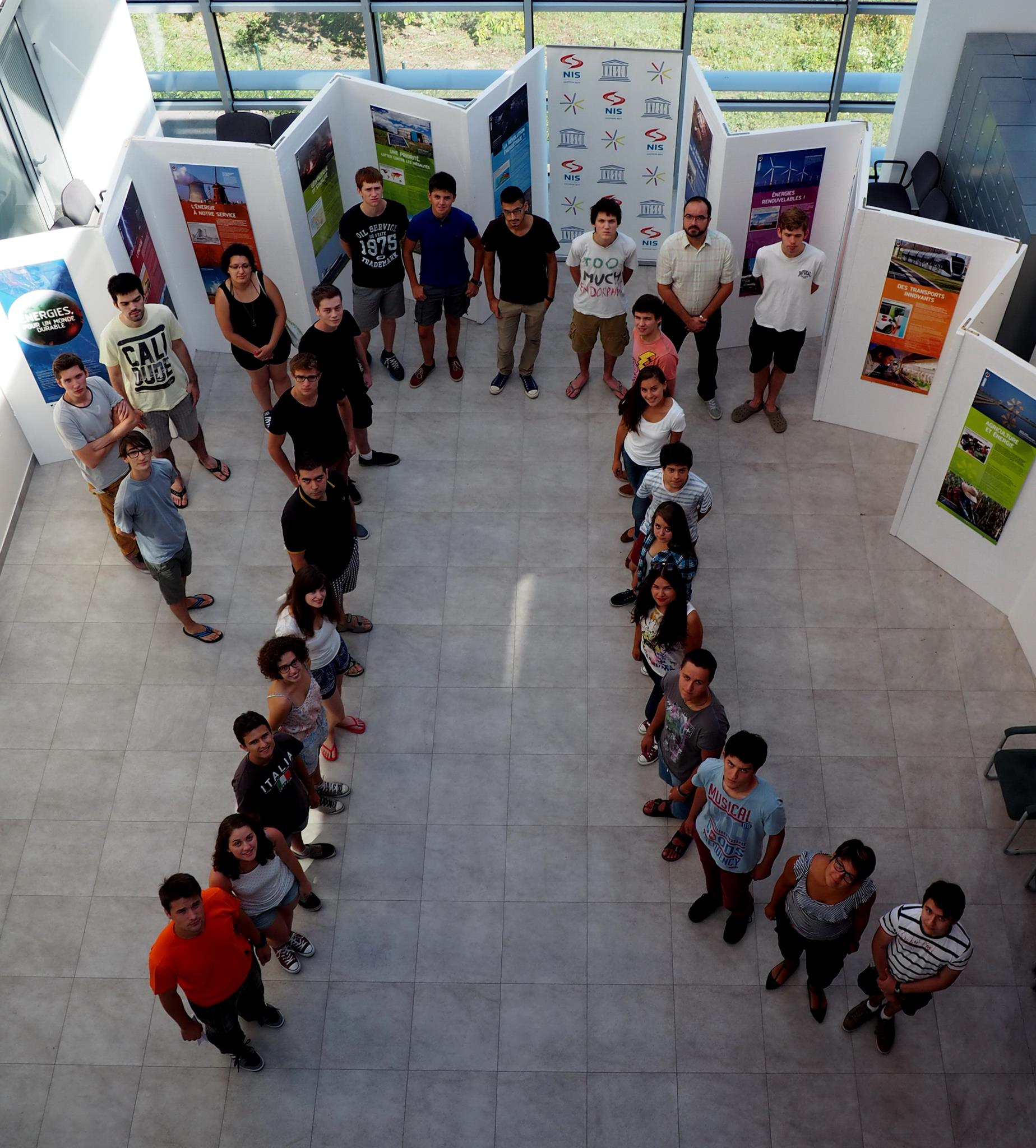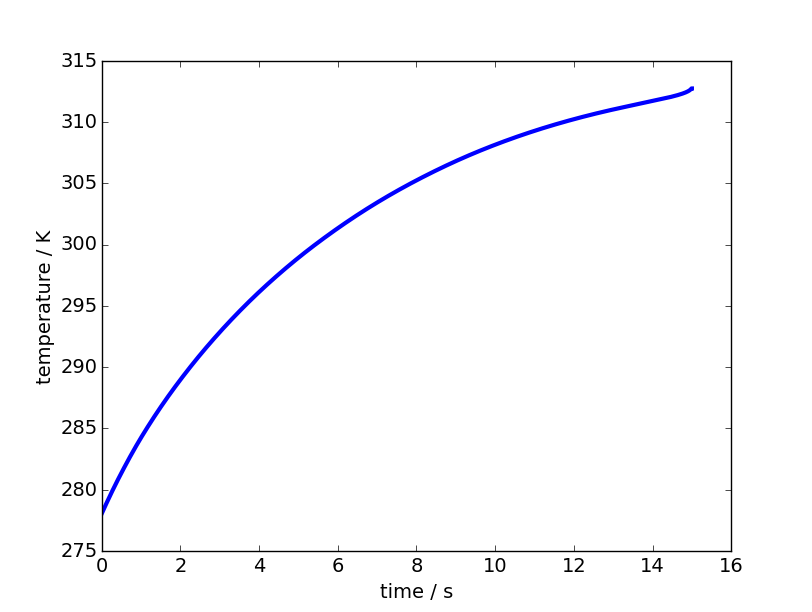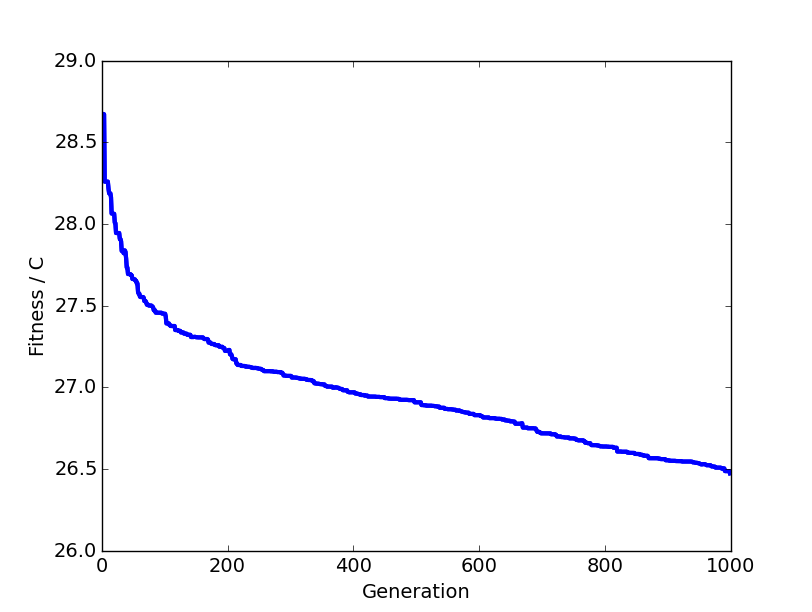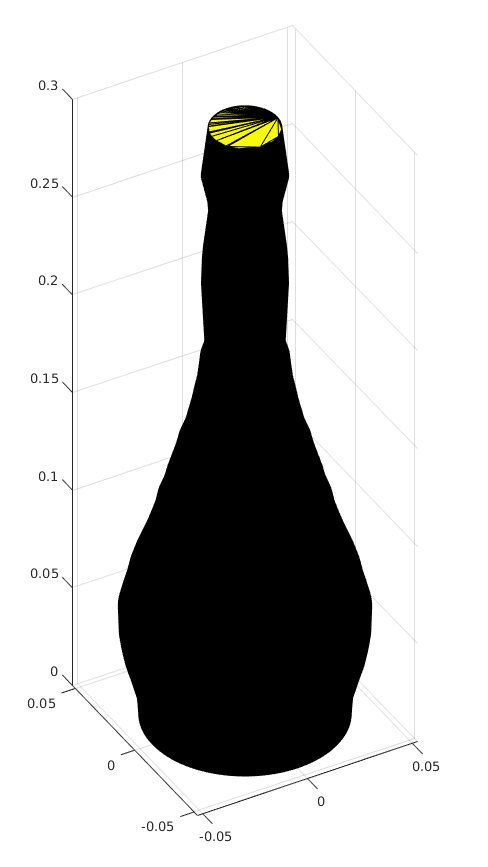# Petnica International and optimizing glass shapes

Over the last 10 days I’ve been at Petnica International, a science seminar in Serbia, and it was an incredible experience (not unlike other Petnica programs) - I’ve met lots of cool and interesting people from all over Europe, learned some new physics and computer science - but this post is mainly about my project there.The project concerned optimizing shapes of glasses, in order to lower the average temperature of the contained liquid during drinking. The original idea is sourced from here. My results include a lot of noise, but I’d still like to go over the solution since it was an interesting project to work on. (Most of this post is from the paper I wrote at Petnica.)

# Introduction

As a beverage is being drank from a container, its temperature increases and approaches the ambient temperature - decreasing the overall quality of the drinking experience. The goal of this project is to optimize the shape of the container (a glass), so that the mentioned warming of the liquid is minimized. The solution is composed of two major components: the simulation one, which simulates the drinking process for a single glass, and the optimization one, which works with collections of many glasses and the output of the previous component, to actually improve the shapes.

The code is available on GitHub: github.com/corazza/optimize-glass.

# Problem

## Simulation

Several parameters are supplied to the simulation process: glass shape, temperature of the contained liquid, ambient temperature, and drinking time. Drinking is then simulated with that data, and temperature of the liquid is recorded at each moment. At the end of the simulation, mean temperature during drinking is computed, which determines the utility of the glass (glasses with lower mean temperature are deemed fitter).

## Optimization

The direct relationship between the shape and mean temperature is unknown, and it cannot be known deterministically - therefore, a stochastic method which involves simulating many intermediate and potential shapes must be used. The optimization is an iterative process, which relies on simulation at each step.

# Theory and tools

## Newton’s law of coolingThe central equation used for the simulation is Newton’s law of cooling, which determines the heat differential between two mediums (dQ/dt), dependent on material characteristics (h), the heat transfer surface (A), and the difference in temperatures of the mediums (dT).

## Runge-Kutta 4

Fourth-order Runge-Kutta algorithm is a numerical integration method with a high level of correctness. In this project it is used to integrate the heat differentials computed with Newton’s law of cooling.

## Genetic algorithm

Genetic algorithms are a class of stochastic search algorithms inspired by biological evolution. The search space usually has many dimensions, and vectors are analogous to chromosomes in genetics. Each chromosome is evaluated for its fitness (i.e. utility, or mean temperature in this case), and an artificial selection pressure is applied. The selected chromosomes are used to construct the next generation of chromosomes, using genetic operators such as mutation and crossover.

As this process is repeated, the mean and best fitness rise, as those chromosome features which contribute to them are selected with a higher probability.

# Method and implementation

## Chromosome representation

Chromosomes are stored as 50-element arrays of floating point numbers, representing radii at equidistant points of the glass. Each glass has the same total height, and the distances between radii samples (the 50 points) are the same for each glass.

## Evaluation (simulation) and preparation

### Glass interpolation

50 points are not enough to accurately integrate temperature differentials, thus Numpy is used to interpolate the radii of the glass in order to obtain a smoother shape.

### Computing height-dependent characteristics for simulation and why that is not enough

In order to use Newton’s law of cooling, two surfaces must be known at each moment: surface of the top of the liquid, where it touches the air; and the side surface, where it touches the glass.

However, because of the irregular glass shape, the height doesn’t change linearly with time. The value which changes linearly is volume, since drinking speed is assumed constant. This means that the functions height -> top surface and height -> side surface, need to be converted into volume -> top surface and volume -> side surface.

In order to do that, one other function needs to be computed: volume -> height, which can be composed with the previous functions to obtain the desired forms.

### Transformation of f(height) to f(volume)

In order to obtain volume -> height, it is easier to first compute height -> volume, and then compute the inverse using Numpy. Afterwards, the above mentioned composition is used to obtain the functions of volume.

### Simulation (temperature integration)

The simulation is done in N steps, where N depends on total drinking time and the time step, which determines the precision of the simulation.

After the simulation, the mean is computed from the temperatures in each moment.

## Genetic algorithm

The genetic algorithm is ran for some predetermined number of generations of a fixed number of chromosomes, M=100.

### Initialization

In the initialization, the first generation of chromosomes is randomly constructed, each one from three random numbers which are interpolated using spline and sampled at 50 points to arrive at smooth radii.

### Evaluation

Each chromosome in the generation is evaluated for its fitness by computing the mean temperature of its glass. The glasses are compared with the less-than operator determining the better glass, since lower drift in temperature is desired.

### Selection

After evaluation, a new generation must be constructed. First, the previous generation is ranked by fitness. Then the first 1/4th of the ranked previous generation is let through unmodified, in order to ensure propagation of useful features. Then the other 3/4ths are created by crossing over and mutating the mentioned best 1/4ths.

### Crossover and mutation

After selection, parent pairs are crossed over with some adjustable probability, i.e. a child can be created by combining the parent chromosomes. Then, there is a random chance of mutation of each of the child’s radii (which can also be adjusted). The mutation is in the range of -1 to 1 centimeter.

## Parallelization

The evaluation part of the genetic algorithm is parallelized over N cores. Each successive generation is divided into N sections, which are processed independently of each other in parallel. The results are then composed back, and the genetic algorithm continues with the selection.

# Results

## Simulation

The simulation component produces an expected temperature change diagram:• Figure 1: temperature of liquid over time

The result is expected because the differential falls off through time, since it depends on the temperature difference.

## Increase of fitness

The algorithm decreases temperature drift, i.e. the fitness rises. This is evident from the following diagram:• Figure 2: decreasing temperature drift over 1000 generations

The above diagram shows how the difference between the ambient temperature and mean liquid temperature lowers over generation algorithm, which signifies an improvement.

## Produced glasses

After 3600 generations, this was the best glass of the last generation:• Figure 3: 3D render

Although it does look more like a bottle than a glass, I think the majority of the shape does make sense.

## Discussion

The produced glasses have a tendency towards an expected shape (elongated sphere with the volume concentrated at the bottom) - however, the genetic algorithm is simply too slow to converge on an optimal shape. An expected optimal shape would follow a smoother function.

The reason for this most likely lies in the parameters for the genetic algorithm, which determine the exact searching mechanism.